# SAT Math Multiple Choice Question 976: Answer and Explanation

### Test Information

Question: 976

12.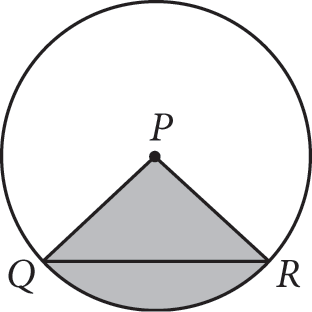The area of the shaded sector in circle P above is 18π square units. If the measure of angle PQR is 45°, what is the length of chord QR?

• A. 6
• B. 9
• C.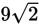• D. 12

Explanation:

D

Difficulty: Hard

Category: Additional Topics in Math / Geometry

Strategic Advice: The area of a sector is found by multiplying the area of the whole circle by the portion of the circle that the sector represents. To find the portion of the circle's area that the shaded sector represents, write the measure of the central angle of the sector over 360°.

Getting to the Answer: This question requires thinking backward to come up with a plan. You need to find the length of chord QR. To find this length, you'll need to know the radius of the circle. To find the radius, you need to find the area of the whole circle by finding the portion of the circle that the shaded sector represents. To do this, you need to know the measure of the central angle of the sector. This is your starting point.

Because PQ and PR are radii, triangle PQR is isosceles. You're given that the measure of angle PQR is 45°, so the measure of angle PRQ is also 45°, making triangle PQR a 45-45-90 triangle. Therefore, the central angle of the shaded sector is 90°, which means the sector represents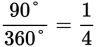of the circle. If the area of this sector is 18π, then the area of the whole circle is 18π × 4 = 72π. Now, use the formula for finding the area of a circle to find the radius: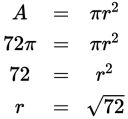Simplify the radical to make the numbers more manageable: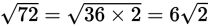. This means each leg of triangle PQR has a length of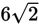. The sides of a 45-45-90 triangle are in the ratio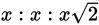, so the hypotenuse of triangle PQR (which is chord QR) has a length of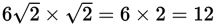. Choice (D) is correct.